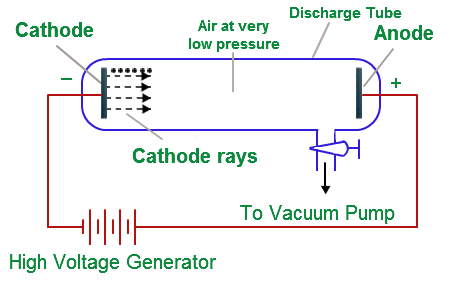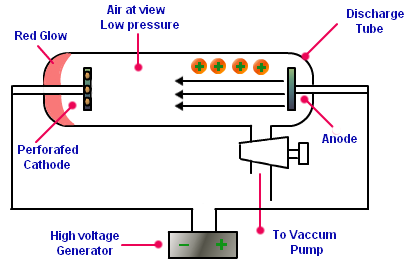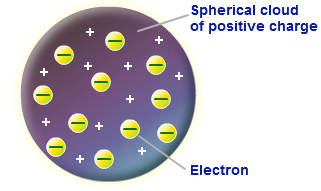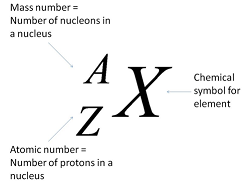# Atomic Structure

In 1803, Dalton’s atomic theory considered the atom to be the smallest indivisible constituent of all matter. The Dalton’s theory could explain the law of conservation of mass, law of constant composition and law of multiple proportions known at that time.

However, towards the end of nineteenth century, certain experiments showed that an atom is neither the smallest nor indivisible particle of matter as stated by Dalton. It was shown to be made up of even smaller particles. These particles were called electrons, protons and neutrons. The electrons are negatively charged whereas the protons are positively charged. The neutrons on the other hand are uncharged in nature.

### Discovery of Electron

In 1885, Sir William Crookes carried out a series of experiments to study the behaviour of metals heated in a vacuum using cathode ray tubes. A cathode ray tube consists of two metal electrodes in a partially evacuated glass tube. An evacuated tube is the one from which most of the air has been removed. The negatively charged electrode is called cathode whereas the positively charged electrode is called anode. These electrodes are connected to a high voltage source.It was observed that when very high voltage was passed across the electrodes in evacuated tube, the cathode produced a stream of particles. These particles were shown to travel from cathode to anode and were called cathode rays.

In 1897, an English physicist Sir J.J. Thomson showed that the rays were made up of a stream of negatively charged particles. This conclusion was drawn from the experimental observations when the experiment was done in the presence of an external electric field.

Properties of Cathode Rays

• Cathode rays travel in straight line
• The particles constituting cathode rays carry mass and possess kinetic energy
• The particles constituting cathode rays have negligible mass but travel very fast
• Cathode ray particles carry negative charge and are attracted towards positively charged plate when an external electric field is applied
• The nature of cathode rays generated was independent of the nature of the gas filled in the cathode ray tube as well as the nature of metal used for making cathode and anode.
• In all the cases the charge to mass ratio (e/m) was found to be the same.

These particles constituting the cathode rays were later called electrons. Since it was observed that the nature of cathode rays was the same irrespective of the metal used for the cathode or the gas filled in the cathode ray tube. This led Thomson to conclude that all atoms must contain electrons.

### Discovery of Proton

Much before the discovery of electron, Eugen Goldstein (in 1886) performed an experiment using a perforated cathode (a cathode having holes in it) in the discharge tube filled with air at a very low pressure. When a high voltage was applied across the electrodes in the discharge tube, a faint red glow was observed behind the perforated cathode.This glow was due to another kind of rays flowing in a direction opposite to that of the cathode rays. These rays were called as anode rays or positive rays. These were positively charged and were also called canal rays because they passed through the holes or the canals present in the perforated cathode.

Properties of Anode Rays

• Like cathode rays, the anode rays also travel in straight lines.
• The particles constituting anode rays carry mass and have kinetic energy.
• The particles constituting canal rays are much heavier than electrons and carry positive charges.
• The positive charge on the particles was whole number multiples of the amount of charge present on the electron.
• The nature and the type of the particles constituting the anode rays were dependent on the gas present in the discharge tube.

The electrons emitted from the cathode collide with the neutral atoms of the gas present in the tube and remove one or more electrons present in them. This leaves behind positive charged particles which travel towards the cathode. When the cathode ray tube contained hydrogen gas, the particles of the canal rays obtained were the lightest and their charge to mass ratio (e/m ratio) was the highest.

Rutherford showed that these particles were identical to the hydrogen ion (hydrogen atom from which one electron has been removed). These particles were named as protons and were shown to be present in all matter.

### Thomson Model

Having discovered electron as a constituent of atom, Thomson concluded that there must be an equal amount of positive charge present in an atom. On this basis he proposed a model for the structure of atom. According to his model, atoms can be considered as a large sphere of uniform positive charge with a number of small negatively charged electrons scattered throughout it. This model was called as plum pudding model.### Rutherford’s Model

Ernest Rutherford and his co-workers were working in the area of radioactivity. They were studying the effect of alpha (α) particles on matter. The alpha particles are helium nuclei, which can be obtained by the removal of two electrons from the helium atom.

α-Ray scattering experiment

In this experiment a stream of α particles from a radioactive source was directed on a thin (about 0.00004 cm thick) piece of gold foil.On the basis of Thomson’s model it was expected that the alpha particles would just pass straight through the gold foil and could be detected by a photographic plate placed behind the foil. However, with the actual results, it was observed that:

• (i) Most of the α-particles passed straight through the gold foil.
• (ii) Some of the α-particles were deflected by small angles.
• (iii) A few particles were deflected by large angles.
• (iv) About 1 in every 12000 particles experienced a rebound.

The results of α-ray scattering experiment were explained by Rutherford in 1911 and another model of the atom was proposed. According to Rutherford’s model:

• An atom contains a dense and positively charged region located at its centre; it was called as nucleus.
• All the positive charge of an atom and most of its mass was contained in the nucleus.
• The rest of an atom must be empty space which contains the much smaller and negatively charged electrons.

The α particles passing through the atom in the region of the electrons would pass straight without any deflection. Only those particles that come in close vicinity of the positively charged nucleus get deviated from their path. Very few α-particles, those that collide with the nucleus, would face a rebound.

Drawbacks of Rutherford's Model

According to Rutherford’s model the negatively charged electrons revolve in circular orbits around the positively charged nucleus. However, according to Maxwell’s electromagnetic theory, if a charged particle accelerates around another charged particle then it would continuously lose energy in the form of radiation. Rutherford's model is unable to explain the stability of the atom.

The Rutherford’s model also does not say anything about the way the electrons are distributed around the nucleus.

Another drawback of the Rutherford’s model was its inability to explain the relationship between the atomic mass and atomic number (the number of protons). This problem was solved later by Chadwick by discovering neutron, the third particle constituting the atom.

The problem of the stability of the atom and the distribution of electrons in the atom was solved by Neils Bohr by proposing yet another model of the atom.

### Bohr's Model of Atom

In 1913, Niels Bohr proposed a model to account for the shortcomings of Rutherford’s model. The postulates are:

Postulate 1: The electrons move in definite circular paths of fixed energy around a central nucleus.

These paths are called orbits, or energy levels. The electron moving in the orbit does not radiate. In other words,
it does not lose energy; therefore, these orbits are called stationary orbits or stationary states.

It was later realised that the concept of circular orbit as proposed by Bohr was not adequate and it was modified to energy shells with definite energy.Postulate 2: The electron can change its shells or energy level by absorbing or releasing energy. An electron at a lower state of energy Ei can go to a final higher state of energy Ef by absorbing a single photon of energy given by:

E = hν = Ef – Ei

Similarly, when electron changes its shell from a higher initial level of energy Ei to a lower final level of energy Ef, a single photon of energy hν is released.

Thus, the Bohr’s model of atom removes two of the limitations of Rutherford’s model. These are related to the stability of atom and the distribution of electrons around the nucleus.

### Discovery of Neutron

According to the Rutherford’s model, the mass of helium atom (containing 2 protons) should be double that of a hydrogen atom (with only one proton). (Ignoring the mass of electron as it is very light). However, the actual ratio of the masses of helium atom to hydrogen atom is 4:1. It was suggested that there must be one more type of subatomic particle present in the nucleus which may be neutral but have mass.

Such a particle was discovered by James Chadwick in 1932. This was found to be electrically neutral and was named neutron. Neutrons are present in the nucleus of all atoms, except hydrogen. A neutron is represented as n and is found to have a mass slightly higher than that of a proton. Thus, if the helium atom contained 2 protons and 2 neutrons in the nucleus, the mass ratio of helium to hydrogen (4:1) could be explained.

### Atomic Number and Mass Number

The number of protons in an atom is called the atomic number and is denoted by the symbol Z. All atoms of an element have the same atomic number.

In order to account for the electrically neutral nature of the atom, the number of protons in the nucleus is exactly equal to the number of electrons.

Atomic number = number of protons = number of electrons

The number of nucleons in the nucleus of an atom is called its mass number. It is denoted by A and is equal to the total number of protons and neutrons present in the nucleus of an element.

Mass number (A) = number of protons (Z) + number of neutrons (n)### Electronic Configuration

The electrons move in definite paths called orbits or shells around a central nucleus. These orbits or shells have different energies and can accommodate different number of electrons in them. The arrangement of electrons in the various shells or orbits of an atom of the element is known as electronic configuration.

The electron distribution is governed by the following rules:

• These orbits or shells in an atom are represented by the letters K, L, M, N,… or the positive integral numbers, n = 1,2,3,4,….
• The orbits are arranged in the order of increasing energy. The energy of M shell is more than that of the L shell which in turn is more than that of the K shell.
• The maximum number of electrons present in a shell is given by the formula 2n2, where n is the number of the orbit or the shell.
• The shells are occupied in the increasing order of their energies.

• Electrons are not accommodated in a given shell, unless the inner shells are

completely filled.

The maximum number of electrons that can be accommodated in different shells are:

Maximum number of electrons in K shell (or n = 1 level) = 2

Maximum number of electrons in L shell (or n = 2 level) = 8

Maximum number of electrons in M shell (or n = 3 level) = 18

Hydrogen (H) atom has only one electron. It would occupy the first shell and electronic configuration of hydrogen can be represented as 1.

The next element helium (He) has two electrons in its atom. Since the first shell can accommodate two electrons; hence, this second electron will also be placed in the first shell. The electronic configuration of helium is written as 2.

The third element, Lithium (Li) has three electrons. Now the two electrons occupy the first shell whereas the third electron goes to the next shell of higher energy level, i.e. second shell. Thus, the electronic configuration of Li is 2, 1.

### Valency

These electrons in the outermost shell are known as valence electrons. The number of valence electrons determines the combining capacity of an atom in an element. Valence is the number of chemical bonds that an atom can form with univalent atoms. Since hydrogen is a univalent atom, the valence of an element can be taken by the number of atoms of hydrogen with which one atom of the element can combine. For example, in H2O, NH3, and CH4 the valencies of oxygen, nitrogen and carbon are 2, 3 and 4 respectively.

The elements having a completely filled outermost shell in their atoms show little or no chemical activity. In other words, their combining capacity or valency is zero. The elements with completely filled valence shells are said to have stable electronic configuration. The main group elements can have a maximum of eight electrons in their valence shell. This is called octet rule.

How to Calculate Valency

If the number of valence electrons is four or less then the valency is equal to the number of the valence electrons.

Valency = Number of valence electrons (for 4 or lesser valence electrons)

In cases when the number of valence electrons is more than four then generally the valency is equal to 8 minus the number of valence electrons.

Valency = 8 - Number of valence electrons (for more than 4 valence electrons)